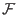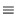Next: Steps to Shor's Algorithm Up: Shor's Algorithm Previous: Introduction to Shor's Algorithm   Contents

## Overview of Shor's Algorithm

Shor's algorithm hinges on a result from number theory. This result is: The function(a) = xa mod n is a periodic function, where x is an integer coprime to n. In the context of Shor's algorithm n will be the number we wish to factor. When two numbers are coprime it means that their greatest common divisor is 1.

Calculating this function for an exponential number of a's would take exponential time on a classical computer. Shor's algorithm utilizes quantum parallelism to perform the exponential number of operations in one step.

The reason why this function is of utility in factoring large numbers is this:

Since(a) is a periodic function, it has some period r. We know that x0 mod n = 1, so xr mod n = 1, and x2r mod n = 1 and so on since the function is periodic.

Given this information and through the following algebraic manipulation:

xr1 mod n

(xr/2)2 = xr1 mod n

(xr/2)2 -10 mod n

and if r is an even number

(xr/2 -1)(xr/2 +1)0 mod n

We can see that the product (xr/2 -1)(xr/2 + 1) is an integer multiple of n, the number to be factored. So long as xr/2 is not equal to + - 1, then at least one of (xr/2 - 1), (xr/2 + 1) must have a nontrivial factor in common with n. So by computing gcd(xr/2 - 1, n), and gcd(xr/2 + 1, n), we will obtain a factor of n, where gcd is the greatest common denominator function.Next: Steps to Shor's Algorithm Up: Shor's Algorithm Previous: Introduction to Shor's Algorithm   Contents
Matthew Hayward - Quantum Computing, Shor's Algorithm, and Parallelism GitHub Repository